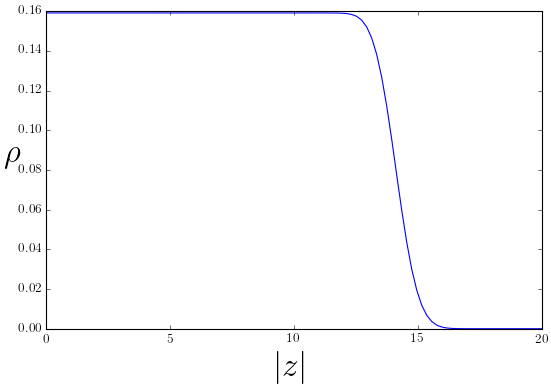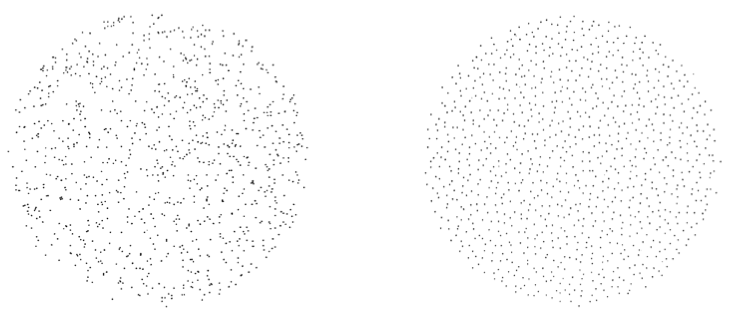# Quantum Hall Effect

• A quantum state in two dimensions

• 2D electron gas in strong perpendicular magnetic fields.

• Can exist for bosons too!

$$\nonumber \newcommand{\br}{\mathbf{r}} \newcommand{\bp}{\mathbf{p}} \newcommand{\bk}{\mathbf{k}} \newcommand{\bq}{\mathbf{q}} \newcommand{\bv}{\mathbf{v}} \newcommand{\pop}{\psi^{\vphantom{\dagger}}} \newcommand{\pdop}{\psi^\dagger} \newcommand{\Pop}{\Psi^{\vphantom{\dagger}}} \newcommand{\Pdop}{\Psi^\dagger} \newcommand{\Phop}{\Phi^{\vphantom{\dagger}}} \newcommand{\Phdop}{\Phi^\dagger} \newcommand{\phop}{\phi^{\vphantom{\dagger}}} \newcommand{\phdop}{\phi^\dagger} \newcommand{\aop}{a^{\vphantom{\dagger}}} \newcommand{\adop}{a^\dagger} \newcommand{\bop}{b^{\vphantom{\dagger}}} \newcommand{\bdop}{b^\dagger} \newcommand{\cop}{c^{\vphantom{\dagger}}} \newcommand{\cdop}{c^\dagger} \newcommand{\bra}{\langle{#1}\rvert} \newcommand{\ket}{\lvert{#1}\rangle} \newcommand{\inner}{\langle{#1}\rvert #2 \rangle} \newcommand{\braket}{\langle{#1}\rvert #2 \lvert #3 \rangle} \newcommand{\sgn}{\mathrm{sgn}} \DeclareMathOperator{\tr}{tr} \DeclareMathOperator{\E}{\mathbb{E}} \newcommand{\brN}{\br_1, \ldots, \br_N}$$

## Landau Levels

• Single particle Hamiltonian with vector potential

$$H = -\frac{1}{2m}\left(\nabla -i q \mathbf{A}\right)^2, \label{Landau}$$

• For perpendicular field

$$\partial_x A_y - \partial_y A_x = B.$$

• We choose symmetric gauge

$$A_x = -\frac{1}{2} B y,\quad A_y = \frac{1}{2} B x.$$

## $H$ in complex coordinates

\begin{align} z = x + i y \quad \bar z = x - iy,\\ \partial_z = \frac{1}{2}\left(\partial_x - i\partial_y\right) \quad \partial_{\bar z} = \frac{1}{2}\left(\partial_x + i\partial_y\right).\\ H = -\frac{2}{m}\left(\partial_z -\frac{qB \bar z}{4}\right)\left(\partial_{\bar z} +\frac{qB z}{4}\right) + \frac{\omega_c}{2} \end{align}

• $\omega_c = \frac{qB}{m}$ is the cyclotron frequency

• States that satisfy $$\left(\partial_{\bar z} +\frac{qB z}{4}\right)\psi(z,\bar z) = 0$$ have energy $\omega_c/2$ and belong to the lowest Landau level (LLL).

Show that these states have the form

$$\psi(z,\bar z) = f(z) \exp\left(-\frac{qB}{4}\left|z\right|^2\right),$$

where $f(z)$ is an arbitrary analytic function.

• $f(z)=a_0 + a_1z + a_2 z^2+\ldots$ for arbitrary $a_n$

• Landau levels highly degenerate

## Notation

• Write LLL wavefunction as $f(z)$, leaving Gaussian in inner product

$$\bra{f_1}f_2\rangle = \int \frac{d^2z}{2\pi} \overline{f_1(z)}f_2(z) \exp\left(-\left|z\right|^2/2\right),$$

• Units with magnetic length $\ell \equiv (qB)^{-1/2}=1$

• A possible orthonormal basis is $f_n(z) = \frac{z^n}{\sqrt{2^n n!}}$

Show this

## Filled LLL of Fermions

• Lift degeneracy by adding harmonic potential $$V_\text{harm}(x,y) = \frac{v}{2}\left(x^2+y^2\right) = \frac{v}{2}\left|z\right|^2. \label{many_HarmonicRound}$$

• Problem: $V_\text{harm}(z,\bar z)f(z)$ is not in LLL

• Consider only the action of $V$ in the LLL subspace.

By considering matrix elements $\bra{f_1}V\ket{f_2}$ between LLL states, show (by integrating by parts) that it is possible to replace any occurrence of $\bar z$ in $V$ with $2\partial_z$ acting on the analytic part of the wavefunction.

Note that the order is important: all the $\partial_z$ must stand to the left of the $z$, Thus $$V_\text{harm}\longrightarrow v\partial_z z = v\left(1+z \partial_z\right). \label{many_HarmonicProject}$$

• Applied to $f_n(z)$, $V$ just counts the degree: $V_\text{harm} f_n = v(1+n)f_n$.
• Fill states $n=0,1,\ldots N-1$

• As for 1D fermions, single particle states are powers $z^n$.

• Identical arguments imply

$$\Psi(z_1,\ldots, z_N) = \prod_{j<k}^N (z_j-z_k) \exp\left(-\frac{1}{4}\sum_{j=1}^N\left|z_j\right|^2\right) \label{many_nu1}$$

Show that the density is $$\rho_1(z,\bar z) = \frac{e^{-|z|^2/2}}{2\pi}\sum_{n=0}^{N-1} \frac{\left|z\right|^{2n}}{2^n n!} = \frac{1}{2\pi} \frac{\Gamma(N,|z|^2/2)}{(N-1)!}. \label{many_LLLdensity}$$ $\Gamma(s,x) = \int^\infty_x t^{s-1}e^{-t}dt$ is incomplete gamma fn.• At small $\left|z\right|$, approximate by infinite sum, and $\rho_1\to \frac{1}{2\pi}$

• The density is fixed at this value until we reach $\sim\sqrt{2N}$, then falls to zero on scale of $\ell_B$.

• The filled LLL is described by a circular droplet of fixed density $\rho_1 = 1/(2\pi)$: one state per flux quantum $\Phi_0=2\pi/q$

• Changing confining potential causes the droplet to deform, but density to remain constant

## The Laughlin Wavefunction

• In 1983 Robert Laughlin proposed the generalization $$\Psi_m(z_1,\ldots, z_N) = \prod_{j<k}^N (z_j-z_k)^{m} \exp\left(-\frac{1}{4}\sum_{j=1}^N\left|z_j\right|^2\right). \label{many_nu}$$

• $m$ odd / even for fermions / bosons. $m\neq 1$ not a product state!

This Letter presents variational ground-state and excited-state wave functions which describe the condensation of a two-dimensional electron gas into a new state of matter.

## $m=2$ boson state

• With the repulsive potential $$H_{\text{int}} = g\sum_{j<k}\delta(\br_j-\br_k),\qquad g>0 \label{many_delta}$$ $m=2$ state has zero interaction energy

• Any state with zero interaction energy must have $\Psi_2(z_1,\ldots, z_N)$ as a factor

• If wavefunction has higher degree, then in the presence of potential $V_\text{harm}$ it will have higher energy, so $\Psi_2$ is ground state

## Particle distribution• Monte Carlo samples from uncorrelated (uniform) distrubution vs. the Laughlin probability distribution $\lvert\Psi_3(z_1,\ldots z_N)\rvert^2$.

• Note uniformity of particle distribution, in contrast with the sample of uncorrelated particles on the left

## The Plasma Analogy

• Probability distribution $\lvert\Psi_m(z_1,\ldots, z_N)\rvert^2$ of particles interpreted as Boltzmann distribution of particles in a classical 2D plasma

$$\nabla^2 V = -q\delta(\br).$$

• In 2D the solution describing a point charge is $$V_\text{point}(\br) = -\frac{q}{2\pi}\log\left|\br\right|,$$
• Constant background charge density $-\rho_0$ gives rise to a potential $$V_\text{bg}(\br) = \frac{\rho_0}{4} \left|\br\right|^2.$$
• System of identical charges in a background charge has overall electrostatic energy $$V(\br_1,\ldots,\br_N) = -\frac{q^2}{2\pi} \sum_{j<k}\log\left|\br_j-\br_k\right| + \frac{q\rho_0}{4}\sum_j \left|\br_j\right|^2.$$

• At finite temperature (unnormalized) probability of finding particles at locations $\br_1, \ldots, \br_N$ is $$\exp[-\beta V(\brN)] = \left|\Psi_m(\brN)\right|^2,$$ where we set $\beta q^2/(2\pi) = 2m$ and $\beta q\rho_0 = 2$.

• These are not physical electrostatic fields!

## $N\to\infty$ limit

• Introduce a continuum charge density $\rho(\br)$ and write the energy as a functional \begin{align} \beta V[\rho] = -m\int d^2\br\, d^2\br'\rho(\br)\log\left|\br-\br'\right|\rho(\br') + \frac{1}{2}\int d^2\br\left|\br\right|^2\rho(\br). \end{align}

Show that minimizing the energy with respect to $\rho(\br)$ – corresponding to finding the most likely configuration – leads to the condition $$-2m\int d^2\br’\log\left|\br-\br’\right|\rho(\br’) + \frac{1}{2}\left|\br\right|^2 = 0.$$

Show that applying $\nabla^2$ to both sides gives $$\rho(\br) = \frac{1}{2\pi m}.$$

• Conclusion: density is fixed at $1/m$ of value for $m=1$ case

• Result applies where density is non-zero, so we get a uniform droplet of radius $\sqrt{2Nm}$

• $1/m$ is called the filling fraction of the state.

## Excited states: fractional charge

• Simplest excited state is quasihole \begin{align} \Psi_\text{hole}(z_1,\ldots, z_N|Z) &= \left(\prod_j (Z-z_j)\right)\Psi_m(z_1,\ldots, z_N)\\ V(\brN)&=-\frac{q^2}{2\pi m}\sum_j \log\left|\br_j-\mathbf{R}\right|-\frac{q^2}{2\pi} \sum_{j<k}\log\left|\br_j-\br_k\right|\\ &+ \frac{\rho q_0}{4}\sum_j \left|\br_j\right|^2. \end{align}

• Charge $q/m$ at point $\mathbf{R} = (X, Y)$, where $Z=X+iY$

• Plasma will screen this charge, leaving a ‘hole’ in density distribution of charge $-q/m$

## Hole state

• 20 holes with charge $-20/m$ for clarity

## Fractional Statistics

• Two quasihole wavefunction

$$\Psi_\text{2 hole}(z_1,\ldots, z_N|Z_1,Z_2) = \left(\prod_j (Z_1-z_j)(Z_2-z_j)\right)\Psi_m(z_1,\ldots, z_N).$$

• $\vert\Psi_\text{2 hole}(z_1,\ldots, z_N\vert Z_1,Z_2)\rvert^2$ is plasma with two $1/m$ charges at positions $\mathbf{R}_{1,2}$

• Each surrounded by depleted density of $-1/m$ of a particle

• (See notes) Quasiholes are anyons, particles with fractional statistics!